# Chap02-A.pdf

30 May 2023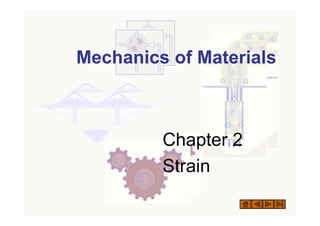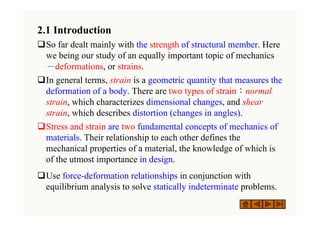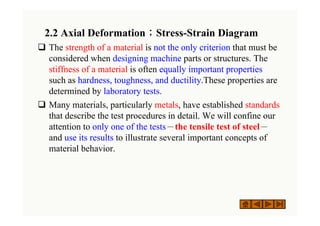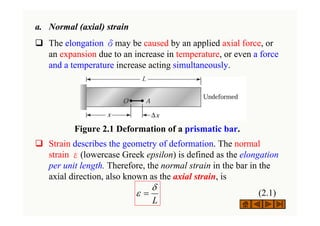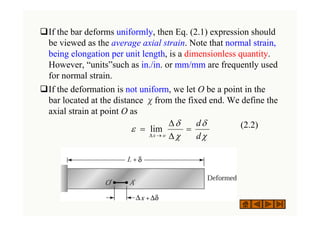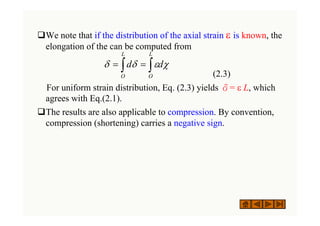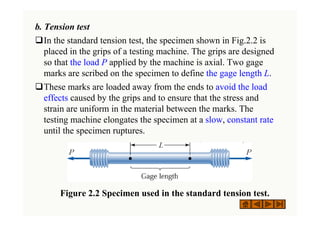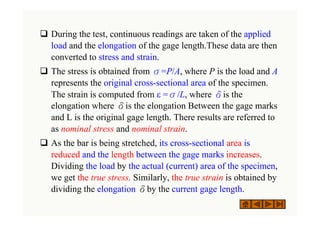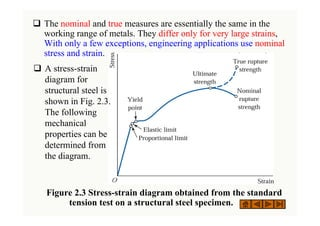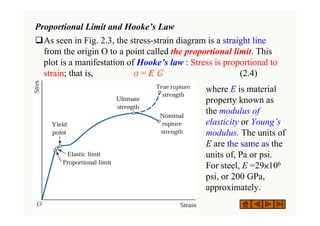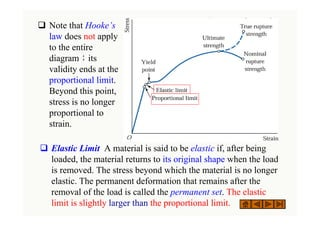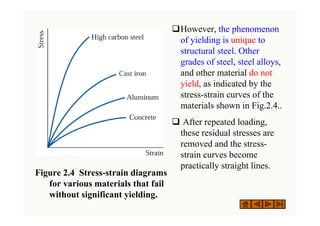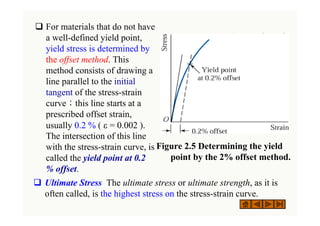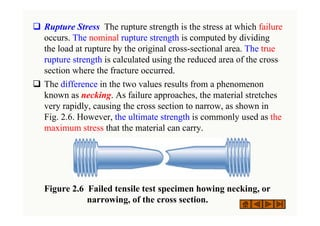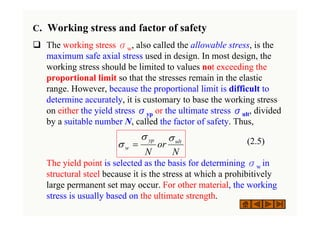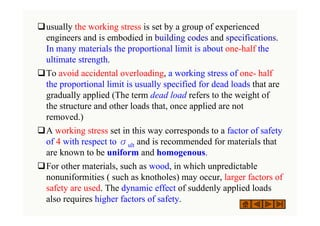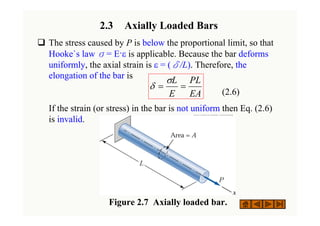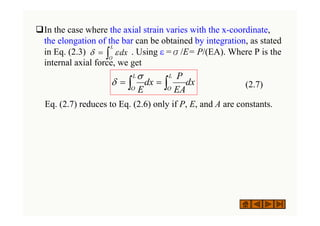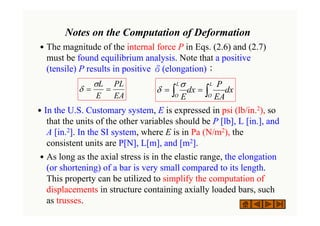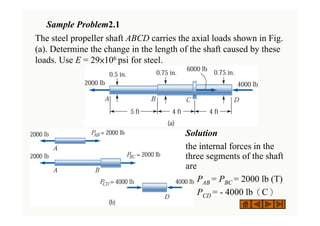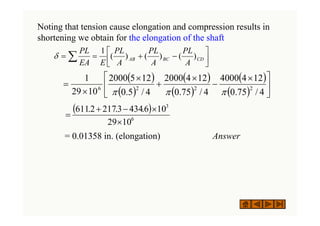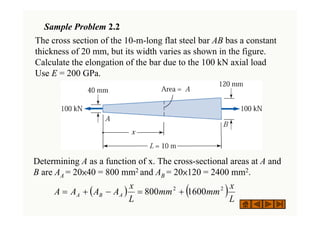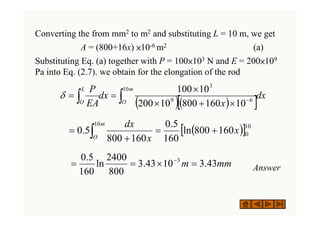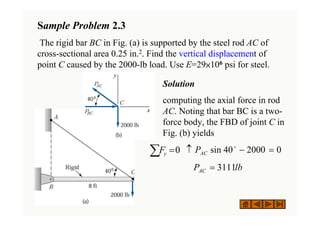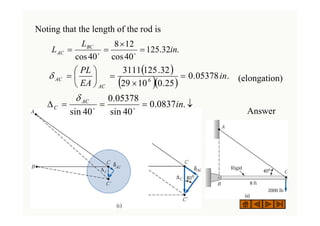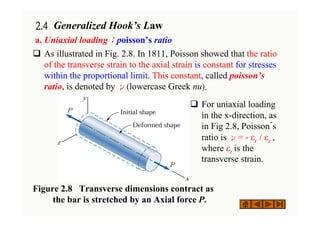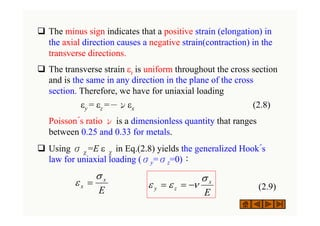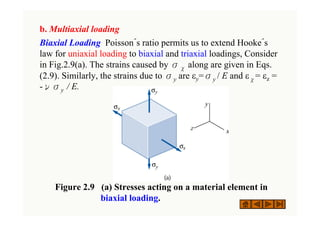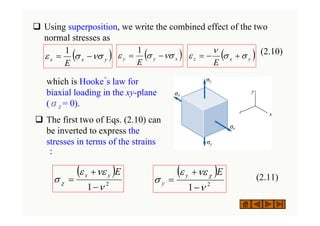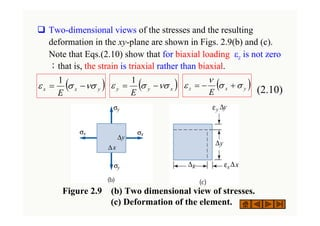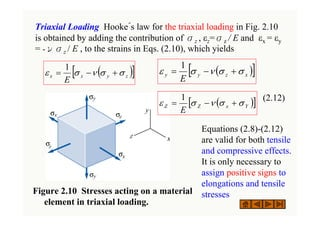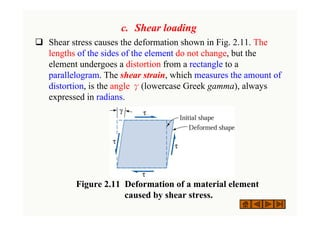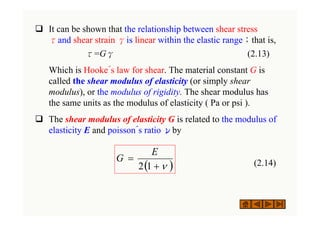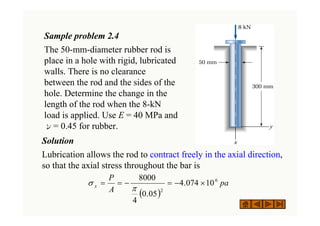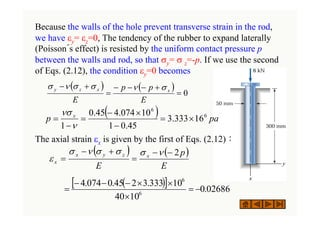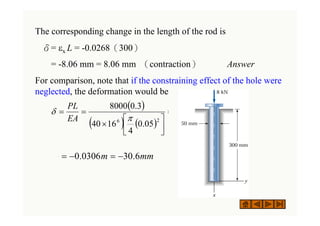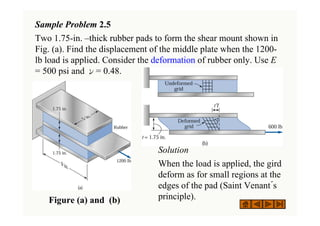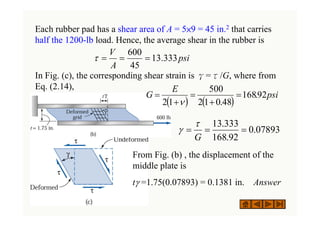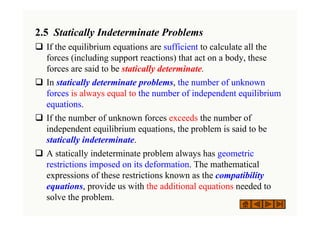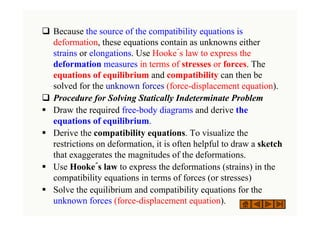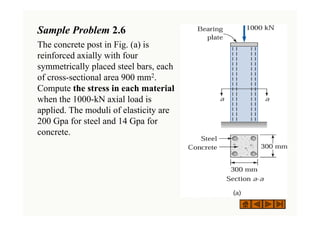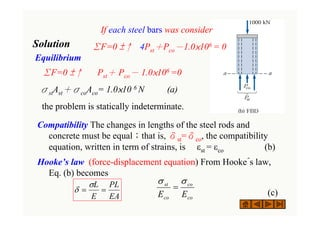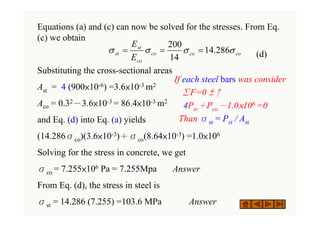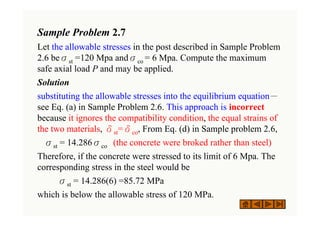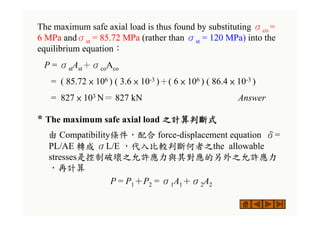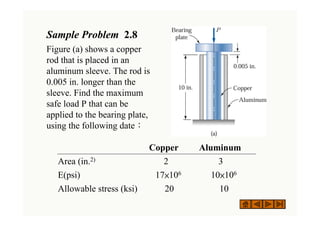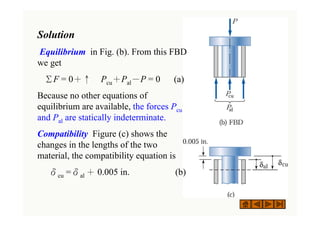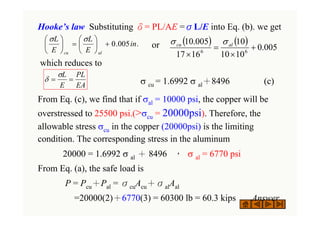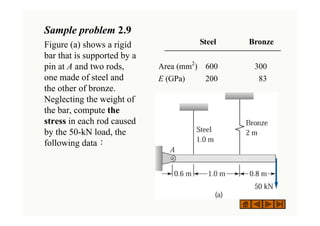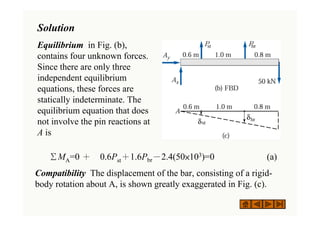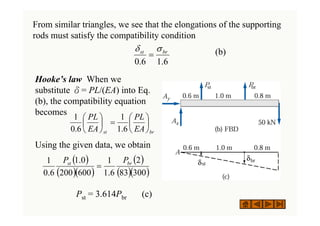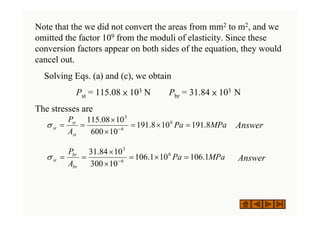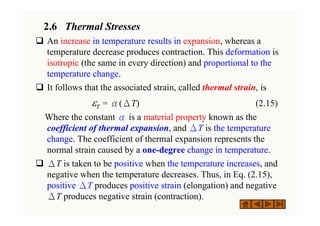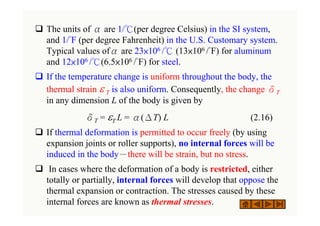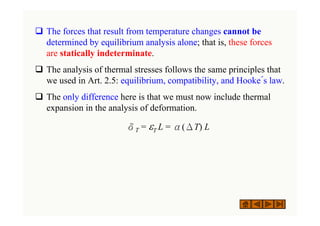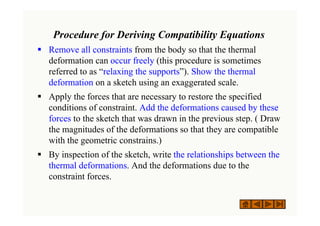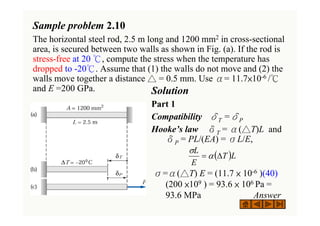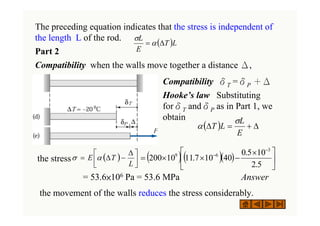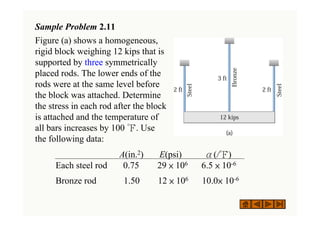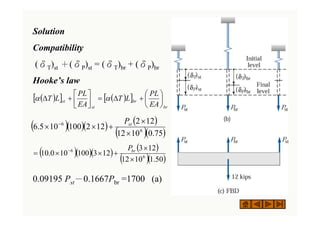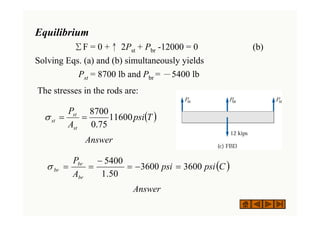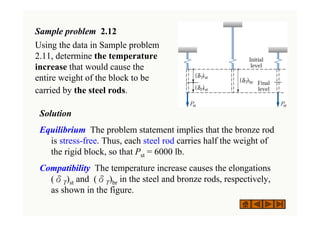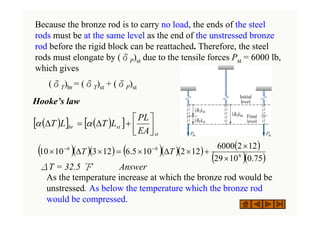1 sur 64

### Chap02-A.pdf

• 2. 2.1 Introduction ‰So far dealt mainly with the strength of structural member. Here we being our study of an equally important topic of mechanics －deformations, or strains. ‰In general terms, strain is a geometric quantity that measures the deformation of a body. There are two types of strain：normal strain, which characterizes dimensional changes, and shear strain, which describes distortion (changes in angles). ‰Stress and strain are two fundamental concepts of mechanics of materials. Their relationship to each other defines the mechanical properties of a material, the knowledge of which is of the utmost importance in design. ‰Use force-deformation relationships in conjunction with equilibrium analysis to solve statically indeterminate problems.
• 3. 2.2 Axial Deformation；Stress-Strain Diagram ‰ The strength of a material is not the only criterion that must be considered when designing machine parts or structures. The stiffness of a material is often equally important properties such as hardness, toughness, and ductility.These properties are determined by laboratory tests. ‰ Many materials, particularly metals, have established standards that describe the test procedures in detail. We will confine our attention to only one of the tests－the tensile test of steel－ and use its results to illustrate several important concepts of material behavior.
• 4. a. Normal (axial) strain ‰ The elongation δmay be caused by an applied axial force, or an expansion due to an increase in temperature, or even a force and a temperature increase acting simultaneously. ‰ Strain describes the geometry of deformation. The normal strain ε(lowercase Greek epsilon) is defined as the elongation per unit length. Therefore, the normal strain in the bar in the axial direction, also known as the axial strain, is (2.1) L δ ε = Figure 2.1 Deformation of a prismatic bar.
• 5. ‰If the bar deforms uniformly, then Eq. (2.1) expression should be viewed as the average axial strain. Note that normal strain, being elongation per unit length, is a dimensionless quantity. However, “units”such as in./in. or mm/mm are frequently used for normal strain. ‰If the deformation is not uniform, we let O be a point in the bar located at the distance χfrom the fixed end. We define the axial strain at point O as (2.2) χ δ χ δ ε d d o x = Δ Δ = → Δ lim
• 6. ‰We note that if the distribution of the axial strain ε is known, the elongation of the can be computed from (2.3) For uniform strain distribution, Eq. (2.3) yields δ= ε L, which agrees with Eq.(2.1). ‰The results are also applicable to compression. By convention, compression (shortening) carries a negative sign. ∫ ∫ = = L O L O d d χ ε δ δ
• 7. b. Tension test ‰In the standard tension test, the specimen shown in Fig.2.2 is placed in the grips of a testing machine. The grips are designed so that the load P applied by the machine is axial. Two gage marks are scribed on the specimen to define the gage length L. ‰These marks are loaded away from the ends to avoid the load effects caused by the grips and to ensure that the stress and strain are uniform in the material between the marks. The testing machine elongates the specimen at a slow, constant rate until the specimen ruptures. ( ) g ( ) g Figure 2.2 Specimen used in the standard tension test.
• 8. ‰ During the test, continuous readings are taken of the applied load and the elongation of the gage length.These data are then converted to stress and strain. ‰ The stress is obtained from σ=P/A, where P is the load and A represents the original cross-sectional area of the specimen. The strain is computed from ε =σ/L, where δis the elongation where δis the elongation Between the gage marks and L is the original gage length. There results are referred to as nominal stress and nominal strain. ‰ As the bar is being stretched, its cross-sectional area is reduced and the length between the gage marks increases. Dividing the load by the actual (current) area of the specimen, we get the true stress. Similarly, the true strain is obtained by dividing the elongation δby the current gage length.
• 9. ‰ The nominal and true measures are essentially the same in the working range of metals. They differ only for very large strains, With only a few exceptions, engineering applications use nominal stress and strain. ( ) g g ( ) g g Figure 2.3 Stress-strain diagram obtained from the standard tension test on a structural steel specimen. ‰ A stress-strain diagram for structural steel is shown in Fig. 2.3. The following mechanical properties can be determined from the diagram.
• 10. Proportional Limit and Hooke’s Law ‰As seen in Fig. 2.3, the stress-strain diagram is a straight line from the origin O to a point called the proportional limit. This plot is a manifestation of Hooke’s law : Stress is proportional to strain; that is, σ= E Є (2.4) where E is material property known as the modulus of elasticity or Young’s modulus. The units of E are the same as the units of, Pa or psi. For steel, E =29×106 psi, or 200 GPa, approximately.
• 11. ‰ Note that Hooke’s law does not apply to the entire diagram；its validity ends at the proportional limit. Beyond this point, stress is no longer proportional to strain. ( ) g g ( ) g g ‰ Elastic Limit A material is said to be elastic if, after being loaded, the material returns to its original shape when the load is removed. The stress beyond which the material is no longer elastic. The permanent deformation that remains after the removal of the load is called the permanent set. The elastic limit is slightly larger than the proportional limit.
• 12. ‰ Yield Point The point where the stress-strain diagram becomes almost horizontal is called the yield point, and the corresponding stress is known as the yield stress or yield strength. ( ) g g ( ) g g ‰ Beyond the yield point there is an appreciable elongation of the material without a corresponding increase in load. Indeed, the load may actually decrease while the yielding occurs.
• 13. Figure 2.4 Stress-strain diagrams for various materials that fail without significant yielding. ( ) 2003 k /C l bli hi / h i ( ) 2003 k /C l bli hi / h i ‰However, the phenomenon of yielding is unique to structural steel. Other grades of steel, steel alloys, and other material do not yield, as indicated by the stress-strain curves of the materials shown in Fig.2.4.. ‰ After repeated loading, these residual stresses are removed and the stress- strain curves become practically straight lines.
• 14. ‰ For materials that do not have a well-defined yield point, yield stress is determined by the offset method. This method consists of drawing a line parallel to the initial tangent of the stress-strain curve；this line starts at a prescribed offset strain, usually 0.2 % ( ε = 0.002 ). The intersection of this line with the stress-strain curve, is called the yield point at 0.2 % offset. ( ) g g ( ) g g Figure 2.5 Determining the yield point by the 2% offset method. ‰ Ultimate Stress The ultimate stress or ultimate strength, as it is often called, is the highest stress on the stress-strain curve.
• 15. ‰ Rupture Stress The rupture strength is the stress at which failure occurs. The nominal rupture strength is computed by dividing the load at rupture by the original cross-sectional area. The true rupture strength is calculated using the reduced area of the cross section where the fracture occurred. ‰ The difference in the two values results from a phenomenon known as necking. As failure approaches, the material stretches very rapidly, causing the cross section to narrow, as shown in Fig. 2.6. However, the ultimate strength is commonly used as the maximum stress that the material can carry. Figure 2.6 Failed tensile test specimen howing necking, or narrowing, of the cross section.
• 16. C. Working stress and factor of safety ‰ The working stress σw, also called the allowable stress, is the maximum safe axial stress used in design. In most design, the working stress should be limited to values not exceeding the proportional limit so that the stresses remain in the elastic range. However, because the proportional limit is difficult to determine accurately, it is customary to base the working stress on either the yield stress σyp or the ultimate stress σult, divided by a suitable number N, called the factor of safety. Thus, (2.5) The yield point is selected as the basis for determining σw in structural steel because it is the stress at which a prohibitively large permanent set may occur. For other material, the working stress is usually based on the ultimate strength. N or N ult yp w σ σ σ =
• 17. ‰usually the working stress is set by a group of experienced engineers and is embodied in building codes and specifications. In many materials the proportional limit is about one-half the ultimate strength. ‰To avoid accidental overloading, a working stress of one- half the proportional limit is usually specified for dead loads that are gradually applied (The term dead load refers to the weight of the structure and other loads that, once applied are not removed.) ‰A working stress set in this way corresponds to a factor of safety of 4 with respect to σult and is recommended for materials that are known to be uniform and homogenous. ‰For other materials, such as wood, in which unpredictable nonuniformities ( such as knotholes) may occur, larger factors of safety are used. The dynamic effect of suddenly applied loads also requires higher factors of safety.
• 18. 2.3 Axially Loaded Bars ‰ The stress caused by P is below the proportional limit, so that Hooke`s law σ= E·ε is applicable. Because the bar deforms uniformly, the axial strain is ε = (δ/L). Therefore, the elongation of the bar is (2.6) If the strain (or stress) in the bar is not uniform then Eq. (2.6) is invalid. (c) 2003 Brooks/Cole Publishing / Thomson Learning (c) 2003 Brooks/Cole Publishing / Thomson Learning EA PL E L = = σ δ Figure 2.7 Axially loaded bar.
• 19. ‰In the case where the axial strain varies with the x-coordinate, the elongation of the bar can be obtained by integration, as stated in Eq. (2.3) . Using ε =σ/E= P/(EA). Where P is the internal axial force, we get (2.7) Eq. (2.7) reduces to Eq. (2.6) only if P, E, and A are constants. dx L O ∫ = ε δ dx EA P dx E L O L O ∫ ∫ = = σ δ
• 20. Notes on the Computation of Deformation ˙The magnitude of the internal force P in Eqs. (2.6) and (2.7) must be found equilibrium analysis. Note that a positive (tensile) P results in positive δ(elongation)； ˙In the U.S. Customary system, E is expressed in psi (lb/in.2), so that the units of the other variables should be P [lb], L [in.], and A [in.2]. In the SI system, where E is in Pa (N/m2), the consistent units are P[N], L[m], and [m2]. ˙As long as the axial stress is in the elastic range, the elongation (or shortening) of a bar is very small compared to its length. This property can be utilized to simplify the computation of displacements in structure containing axially loaded bars, such as trusses. EA PL E L = = σ δ dx EA P dx E L O L O ∫ ∫ = = σ δ
• 21. Sample Problem2.1 The steel propeller shaft ABCD carries the axial loads shown in Fig. (a). Determine the change in the length of the shaft caused by these loads. Use E = 29×106 psi for steel. ( ) k /C l bli hi / h i ( ) k /C l bli hi / h i Solution the internal forces in the three segments of the shaft are PAB = PBC = 2000 lb (T) PCD = - 4000 lb（C）
• 22. Noting that tension cause elongation and compression results in shortening we obtain for the elongation of the shaft = 0.01358 in. (elongation) Answer ( ) ( ) ( ) ( ) ( ) ( ) ⎥ ⎦ ⎤ ⎢ ⎣ ⎡ × − × + × × = 4 / 75 . 0 12 4 4000 4 / 75 . 0 12 4 2000 4 / 5 . 0 12 5 2000 10 29 1 2 2 2 6 π π π ( ) 6 3 10 29 10 6 . 434 3 . 217 2 . 611 × × − + = ⎥ ⎦ ⎤ ⎢ ⎣ ⎡ − + = = ∑ CD BC AB A PL A PL A PL E EA PL ) ( ) ( ) ( 1 δ
• 23. Sample Problem 2.2 The cross section of the 10-m-long flat steel bar AB bas a constant thickness of 20 mm, but its width varies as shown in the figure. Calculate the elongation of the bar due to the 100 kN axial load Use E = 200 GPa. Determining A as a function of x. The cross-sectional areas at A and B are AA = 20×40 = 800 mm2 and AB = 20×120 = 2400 mm2. ( ) ( )L x mm mm L x A A A A A B A 2 2 1600 800 + = − + =
• 24. Converting the from mm2 to m2 and substituting L = 10 m, we get A = (800+16x) ×10-6 m2 (a) Substituting Eq. (a) together with P = 100×103 N and E = 200×109 Pa into Eq. (2.7). we obtain for the elongation of the rod Answer ( )( ) [ ]dx x dx EA P m O L O ∫ ∫ − × + × × = = 10 6 9 3 10 160 800 10 200 10 100 δ ( ) [ ]10 0 10 160 800 ln 160 5 . 0 160 800 5 . 0 x x dx m O + = + = ∫ mm m 43 . 3 10 43 . 3 800 2400 ln 160 5 . 0 3 = × = = −
• 25. Solution computing the axial force in rod AC. Noting that bar BC is a two- force body, the FBD of joint C in Fig. (b) yields Sample Problem 2.3 The rigid bar BC in Fig. (a) is supported by the steel rod AC of cross-sectional area 0.25 in.2. Find the vertical displacement of point C caused by the 2000-lb load. Use E=29×106 psi for steel. 0 2000 40 sin = − ↑ o AC P lb PAC 3111 = ∑ =0 y F
• 26. Noting that the length of the rod is (elongation) Answer . 32 . 125 40 cos 12 8 40 cos in L L BC AC = × = = o o ( ) ( )( ) . 05378 . 0 25 . 0 10 29 32 . 125 3111 6 in EA PL AC AC = × = ⎟ ⎠ ⎞ ⎜ ⎝ ⎛ = δ ↓ = = = Δ . 0837 . 0 40 sin 05378 . 0 40 sin in AC C o o δ
• 27. 2.4 Generalized Hook’s Law a. Uniaxial loading；poisson’s ratio ‰ As illustrated in Fig. 2.8. In 1811, Poisson showed that the ratio of the transverse strain to the axial strain is constant for stresses within the proportional limit. This constant, called poisson’s ratio, is denoted by ν(lowercase Greek nu). Figure 2.8 Transverse dimensions contract as the bar is stretched by an Axial force P. ‰ For uniaxial loading in the x-direction, as in Fig 2.8, Poisson´s ratio is ν= - εt / εx , where εt is the transverse strain.
• 28. ‰ The minus sign indicates that a positive strain (elongation) in the axial direction causes a negative strain(contraction) in the transverse directions. ‰ The transverse strain εt is uniform throughout the cross section and is the same in any direction in the plane of the cross section. Therefore, we have for uniaxial loading εy = εz =－νεx (2.8) Poisson´s ratio ν is a dimensionless quantity that ranges between 0.25 and 0.33 for metals. ‰ Using σχ=E ε χ in Eq.(2.8) yields the generalized Hook´s law for uniaxial loading (σy=σz=0)： (2.9) E x x σ ε = E x z y σ ν ε ε − = =
• 29. Figure 2.9 (a) Stresses acting on a material element in biaxial loading. b. Multiaxial loading Biaxial Loading Poisson´s ratio permits us to extend Hooke´s law for uniaxial loading to biaxial and triaxial loadings, Consider in Fig.2.9(a). The strains caused by σχ along are given in Eqs. (2.9). Similarly, the strains due to σy are εy=σy / E and εχ= εz = -νσy / E.
• 30. ‰ Using superposition, we write the combined effect of the two normal stresses as (2.10) ( ) y x x E νσ σ ε − = 1 ( ) x y y E νσ σ ε − = 1 ( ) y x z E σ σ ν ε + − = ( ) 2 1 ν νε ε σ χ − + = E y x ( ) 2 1 ν νε ε σ χ − + = E y y which is Hooke´s law for biaxial loading in the xy-plane (σz = 0). ‰ The first two of Eqs. (2.10) can be inverted to express the stresses in terms of the strains ： (2.11)
• 31. Figure 2.9 (b) Two dimensional view of stresses. (c) Deformation of the element. ‰ Two-dimensional views of the stresses and the resulting deformation in the xy-plane are shown in Figs. 2.9(b) and (c). Note that Eqs.(2.10) show that for biaxial loading εz is not zero ；that is, the strain is triaxial rather than biaxial. (2.10) ( ) y x x E νσ σ ε − = 1 ( ) x y y E νσ σ ε − = 1 ( ) y x z E σ σ ν ε + − =
• 32. Triaxial Loading Hooke´s law for the triaxial loading in Fig. 2.10 is obtained by adding the contribution of σz , εz=σz / E and εx = εy = -νσz / E , to the strains in Eqs. (2.10), which yields (2.12) ( ) [ ] z y x x E σ σ ν σ ε + − = 1 ( ) [ ] x z y y E σ σ ν σ ε + − = 1 Figure 2.10 Stresses acting on a material element in triaxial loading. Equations (2.8)-(2.12) are valid for both tensile and compressive effects. It is only necessary to assign positive signs to elongations and tensile stresses ( ) [ ] Y x Z Z E σ σ ν σ ε + − = 1
• 33. c. Shear loading ‰ Shear stress causes the deformation shown in Fig. 2.11. The lengths of the sides of the element do not change, but the element undergoes a distortion from a rectangle to a parallelogram. The shear strain, which measures the amount of distortion, is the angle γ(lowercase Greek gamma), always expressed in radians. Figure 2.11 Deformation of a material element caused by shear stress.
• 34. ‰ It can be shown that the relationship between shear stress τand shear strain γis linear within the elastic range；that is, τ=Gγ (2.13) Which is Hooke´s law for shear. The material constant G is called the shear modulus of elasticity (or simply shear modulus), or the modulus of rigidity. The shear modulus has the same units as the modulus of elasticity ( Pa or psi ). ‰ The shear modulus of elasticity G is related to the modulus of elasticity E and poisson´s ratio νby (2.14) ( ) ν + = 1 2 E G
• 35. Sample problem 2.4 The 50-mm-diameter rubber rod is place in a hole with rigid, lubricated walls. There is no clearance between the rod and the sides of the hole. Determine the change in the length of the rod when the 8-kN load is applied. Use E = 40 MPa and ν= 0.45 for rubber. Solution Lubrication allows the rod to contract freely in the axial direction, so that the axial stress throughout the bar is ( ) pa A P x 6 2 10 074 . 4 05 . 0 4 8000 × − = − = = π σ
• 36. Because the walls of the hole prevent transverse strain in the rod, we have εy= εz=0, The tendency of the rubber to expand laterally (Poisson´s effect) is resisted by the uniform contact pressure p between the walls and rod, so that σy= σ z=-p. If we use the second of Eqs. (2.12), the condition εy=0 becomes The axial strain εx is given by the first of Eqs. (2.12)： ( ) ( ) 0 = + − − − = + − E p p E x x z y σ ν σ σ ν σ ( ) ( ) E p E x z y x x 2 − − = + − = ν σ σ σ ν σ ε ( ) [ ] 02686 . 0 10 40 10 333 . 3 2 45 . 0 074 . 4 6 6 − = × × × − − − = ( ) pa p x 6 6 16 333 . 3 45 . 0 1 10 074 . 4 45 . 0 1 × = − × − = − = ν νσ
• 37. The corresponding change in the length of the rod is δ= εx L = -0.0268（300） = -8.06 mm = 8.06 mm （contraction） Answer For comparison, note that if the constraining effect of the hole were neglected, the deformation would be ( ) ( ) ( ) EA PL 05 . 0 4 16 40 3 . 0 8000 2 6 = ⎥ ⎦ ⎤ ⎢ ⎣ ⎡ × = = π δ mm m 6 . 30 0306 . 0 − = − =
• 38. Sample Problem 2.5 Two 1.75-in. –thick rubber pads to form the shear mount shown in Fig. (a). Find the displacement of the middle plate when the 1200- lb load is applied. Consider the deformation of rubber only. Use E = 500 psi and ν= 0.48. Solution When the load is applied, the gird deform as for small regions at the edges of the pad (Saint Venant´s principle). ( ) k / l bli hi / h i ( ) k / l bli hi / h i Figure (a) and (b)
• 39. Each rubber pad has a shear area of A = 5×9 = 45 in.2 that carries half the 1200-lb load. Hence, the average shear in the rubber is In Fig. (c), the corresponding shear strain is γ=τ/G, where from Eq. (2.14), psi A V 333 . 13 45 600 = = = τ From Fig. (b) , the displacement of the middle plate is tγ =1.75(0.07893) = 0.1381 in. Answer ( ) ( ) psi E G 92 . 168 48 . 0 1 2 500 1 2 = + = + = ν 07893 . 0 92 . 168 333 . 13 = = = G τ γ
• 40. 2.5 Statically Indeterminate Problems ‰ If the equilibrium equations are sufficient to calculate all the forces (including support reactions) that act on a body, these forces are said to be statically determinate. ‰ In statically determinate problems, the number of unknown forces is always equal to the number of independent equilibrium equations. ‰ If the number of unknown forces exceeds the number of independent equilibrium equations, the problem is said to be statically indeterminate. ‰ A statically indeterminate problem always has geometric restrictions imposed on its deformation. The mathematical expressions of these restrictions known as the compatibility equations, provide us with the additional equations needed to solve the problem.
• 41. ‰ Because the source of the compatibility equations is deformation, these equations contain as unknowns either strains or elongations. Use Hooke´s law to express the deformation measures in terms of stresses or forces. The equations of equilibrium and compatibility can then be solved for the unknown forces (force-displacement equation). ‰ Procedure for Solving Statically Indeterminate Problem ƒ Draw the required free-body diagrams and derive the equations of equilibrium. ƒ Derive the compatibility equations. To visualize the restrictions on deformation, it is often helpful to draw a sketch that exaggerates the magnitudes of the deformations. ƒ Use Hooke´s law to express the deformations (strains) in the compatibility equations in terms of forces (or stresses) ƒ Solve the equilibrium and compatibility equations for the unknown forces (force-displacement equation).
• 42. Sample Problem 2.6 The concrete post in Fig. (a) is reinforced axially with four symmetrically placed steel bars, each of cross-sectional area 900 mm2. Compute the stress in each material when the 1000-kN axial load is applied. The moduli of elasticity are 200 Gpa for steel and 14 Gpa for concrete. ( ) 2003 k /C l bli hi / h i ( ) 2003 k /C l bli hi / h i
• 43. Compatibility The changes in lengths of the steel rods and concrete must be equal；that is, δst=δco, the compatibility equation, written in term of strains, is εst = εco (b) Hooke’s law (force-displacement equation) From Hooke´s law, Eq. (b) becomes (c) co co co st E E σ σ = Solution Equilibrium ΣF=0 ±↑ Pst＋ Pco－ 1.0×106 =0 σstAst＋σcoAco= 1.0×10 6 N (a) the problem is statically indeterminate. EA PL E L = = σ δ If each steel bars was consider ΣF=0 ±↑ 4Pst＋Pco－1.0×106 = 0
• 44. Equations (a) and (c) can now be solved for the stresses. From Eq. (c) we obtain (d) Substituting the cross-sectional areas Ast = 4 (900×10-6) =3.6×10-3 m2 Aco = 0.32－3.6×10-3 = 86.4×10-3 m2 and Eq. (d) into Eq. (a) yields (14.286σco)(3.6×10-3)＋σco(8.64×10-3) =1.0×106 Solving for the stress in concrete, we get σco = 7.255×106 Pa = 7.255Mpa Answer From Eq. (d), the stress in steel is σst = 14.286 (7.255) =103.6 MPa Answer co co co co st st E E σ σ σ σ 286 . 14 14 200 = = = If each steel bars was consider ΣF=0 ±↑ 4Pst＋Pco－1.0×106 =0 Than σst = Pst / Ast
• 45. Sample Problem 2.7 Let the allowable stresses in the post described in Sample Problem 2.6 beσst =120 Mpa andσco = 6 Mpa. Compute the maximum safe axial load P and may be applied. Solution substituting the allowable stresses into the equilibrium equation－ see Eq. (a) in Sample Problem 2.6. This approach is incorrect because it ignores the compatibility condition, the equal strains of the two materials, δst=δco, From Eq. (d) in Sample problem 2.6, σst = 14.286σco (the concrete were broked rather than steel) Therefore, if the concrete were stressed to its limit of 6 Mpa. The corresponding stress in the steel would be σst = 14.286(6) =85.72 MPa which is below the allowable stress of 120 MPa.
• 46. The maximum safe axial load is thus found by substituting σco = 6 MPa andσst = 85.72 MPa (rather than σst = 120 MPa) into the equilibrium equation： P = σstAst＋σcoAco = ( 85.72 × 106 ) ( 3.6 × 10-3 )＋( 6 × 106 ) ( 86.4 × 10-3 ) = 827 × 103 N＝ 827 kN Answer * The maximum safe axial load 之計算判斷式 由 Compatibility條件，配合 force-displacement equation δ= PL/AE 轉成 σL/E ，代入比較判斷何者之the allowable stresses是控制破壞之允許應力與其對應的另外之允許應力 ，再計算 P = P1＋P2 = σ1A1＋σ2A2
• 47. Sample Problem 2.8 Figure (a) shows a copper rod that is placed in an aluminum sleeve. The rod is 0.005 in. longer than the sleeve. Find the maximum safe load P that can be applied to the bearing plate, using the following date： Copper Aluminum Area (in.2) 2 3 E(psi) 17×106 10×106 Allowable stress (ksi) 20 10
• 48. Solution Equilibrium in Fig. (b). From this FBD we get ΣF = 0＋↑ Pcu＋Pal－P = 0 (a) Because no other equations of equilibrium are available, the forces Pcu and Pal are statically indeterminate. Compatibility Figure (c) shows the changes in the lengths of the two material, the compatibility equation is δcu =δal ＋ 0.005 in. (b)
• 49. Hooke’s law Substituting δ= PL/AE =σL/E into Eq. (b). we get or which reduces to σ cu = 1.6992 σ al＋8496 (c) From Eq. (c), we find that if σal = 10000 psi, the copper will be overstressed to 25500 psi.(>σcu = 20000psi). Therefore, the allowable stress σcu in the copper (20000psi) is the limiting condition. The corresponding stress in the aluminum 20000 = 1.6992 σ al ＋ 8496 ， σ al = 6770 psi From Eq. (a), the safe load is P = Pcu＋Pal = σcuAcu＋σalAal =20000(2)＋6770(3) = 60300 lb = 60.3 kips Answer . 005 . 0 in E L E L al cu + ⎟ ⎠ ⎞ ⎜ ⎝ ⎛ = ⎟ ⎠ ⎞ ⎜ ⎝ ⎛ σ σ ( ) ( ) 005 . 0 10 10 10 16 17 005 . 10 6 6 + × = × al cu σ σ EA PL E L = = σ δ
• 50. Sample problem 2.9 Figure (a) shows a rigid bar that is supported by a pin at A and two rods, one made of steel and the other of bronze. Neglecting the weight of the bar, compute the stress in each rod caused by the 50-kN load, the following data： Steel Bronze Area (mm2 ) 600 300 E (GPa) 200 83
• 51. Solution Equilibrium in Fig. (b), contains four unknown forces. Since there are only three independent equilibrium equations, these forces are statically indeterminate. The equilibrium equation that does not involve the pin reactions at A is ΣMA=0 ＋ 0.6Pst＋1.6Pbr－2.4(50×103)=0 (a) Compatibility The displacement of the bar, consisting of a rigid- body rotation about A, is shown greatly exaggerated in Fig. (c).
• 52. From similar triangles, we see that the elongations of the supporting rods must satisfy the compatibility condition (b) 6 . 1 6 . 0 br st σ δ = Hooke’s law When we substitute δ= PL/(EA) into Eq. (b), the compatibility equation becomes Using the given data, we obtain Pst = 3.614Pbr (c) br st EA PL EA PL ⎟ ⎠ ⎞ ⎜ ⎝ ⎛ = ⎟ ⎠ ⎞ ⎜ ⎝ ⎛ 6 . 1 1 6 . 0 1 ( ) ( )( ) ( ) ( )( ) 300 83 2 6 . 1 1 600 200 0 . 1 6 . 0 1 br st P P =
• 53. Note that the we did not convert the areas from mm2 to m2, and we omitted the factor 109 from the moduli of elasticity. Since these conversion factors appear on both sides of the equation, they would cancel out. Solving Eqs. (a) and (c), we obtain Pst = 115.08 × 103 N Pbr = 31.84 × 103 N The stresses are Answer Answer MPa Pa A P st st st 8 . 191 10 8 . 191 10 600 10 08 . 115 6 6 3 = × = × × = = − σ MPa Pa A P br br st 1 . 106 10 1 . 106 10 300 10 84 . 31 6 6 3 = × = × × = = − σ
• 54. 2.6 Thermal Stresses ‰ An increase in temperature results in expansion, whereas a temperature decrease produces contraction. This deformation is isotropic (the same in every direction) and proportional to the temperature change. ‰ It follows that the associated strain, called thermal strain, is εT = α(ΔT) (2.15) Where the constant α is a material property known as the coefficient of thermal expansion, and ΔT is the temperature change. The coefficient of thermal expansion represents the normal strain caused by a one-degree change in temperature. ‰ ΔT is taken to be positive when the temperature increases, and negative when the temperature decreases. Thus, in Eq. (2.15), positive ΔT produces positive strain (elongation) and negative ΔT produces negative strain (contraction).
• 55. ‰ The units of α are 1/℃(per degree Celsius) in the SI system, and 1/°F (per degree Fahrenheit) in the U.S. Customary system. Typical values ofα are 23×106 /℃ (13×106 /°F) for aluminum and 12×106 /℃(6.5×106 /°F) for steel. ‰ If the temperature change is uniform throughout the body, the thermal strain ε T is also uniform. Consequently, the change δT in any dimension L of the body is given by δT = εT L = α(ΔT) L (2.16) ‰ If thermal deformation is permitted to occur freely (by using expansion joints or roller supports), no internal forces will be induced in the body－there will be strain, but no stress. ‰ In cases where the deformation of a body is restricted, either totally or partially, internal forces will develop that oppose the thermal expansion or contraction. The stresses caused by these internal forces are known as thermal stresses.
• 56. ‰ The forces that result from temperature changes cannot be determined by equilibrium analysis alone; that is, these forces are statically indeterminate. ‰ The analysis of thermal stresses follows the same principles that we used in Art. 2.5: equilibrium, compatibility, and Hooke´s law. ‰ The only difference here is that we must now include thermal expansion in the analysis of deformation. δT = εT L = α(ΔT) L
• 57. Procedure for Deriving Compatibility Equations ƒ Remove all constraints from the body so that the thermal deformation can occur freely (this procedure is sometimes referred to as “relaxing the supports”). Show the thermal deformation on a sketch using an exaggerated scale. ƒ Apply the forces that are necessary to restore the specified conditions of constraint. Add the deformations caused by these forces to the sketch that was drawn in the previous step. ( Draw the magnitudes of the deformations so that they are compatible with the geometric constrains.) ƒ By inspection of the sketch, write the relationships between the thermal deformations. And the deformations due to the constraint forces.
• 58. Sample problem 2.10 The horizontal steel rod, 2.5 m long and 1200 mm2 in cross-sectional area, is secured between two walls as shown in Fig. (a). If the rod is stress-free at 20 ℃, compute the stress when the temperature has dropped to -20℃. Assume that (1) the walls do not move and (2) the walls move together a distance △ = 0.5 mm. Use α= 11.7×10-6 /℃ and E =200 GPa. Solution Part 1 Compatibility δT =δP Hooke’s law δT = α(△T)L and δP = PL/(EA) = σL/E, σ=α(△T) E = (11.7 × 10-6 )(40) (200 ×109 ) = 93.6 × 106 Pa = 93.6 MPa Answer ( )L T E L Δ = α σ
• 59. The preceding equation indicates that the stress is independent of the length L of the rod. Part 2 Compatibility when the walls move together a distance Δ, Compatibility δT =δP ＋Δ Hooke’s law Substituting forδT andδP as in Part 1, we obtain ( ) Δ + = Δ E L L T σ α the stress = 53.6×106 Pa = 53.6 MPa Answer the movement of the walls reduces the stress considerably. ( ) ⎥ ⎦ ⎤ ⎢ ⎣ ⎡ Δ − Δ = L T E α σ ( ) ( )( ) ⎥ ⎦ ⎤ ⎢ ⎣ ⎡ × − × × = − − 5 . 2 10 5 . 0 40 10 7 . 11 10 200 3 6 9 ( )L T E L Δ = α σ
• 60. Sample Problem 2.11 Figure (a) shows a homogeneous, rigid block weighing 12 kips that is supported by three symmetrically placed rods. The lower ends of the rods were at the same level before the block was attached. Determine the stress in each rod after the block is attached and the temperature of all bars increases by 100 ℉. Use the following data: A(in.2) E(psi) α(/℉) Each steel rod 0.75 29 × 106 6.5 × 10-6 Bronze rod 1.50 12 × 106 10.0× 10-6
• 61. Solution Compatibility (δT)st ＋(δP)st = (δT)br + (δP)br Hooke’s law 0.09195 Pst－0.1667Pbr =1700 (a) ( ) [ ] ( ) [ ] br br st st EA PL L T EA PL L T ⎟ ⎠ ⎞ ⎜ ⎝ ⎛ + Δ = ⎥ ⎦ ⎤ ⎢ ⎣ ⎡ + Δ α α ( )( )( ) ( ) ( )( ) 75 . 0 10 12 12 2 12 2 100 10 5 . 6 6 6 × × + × × − st P ( )( )( ) ( ) ( )( ) 50 . 1 10 12 12 3 12 3 100 10 0 . 10 6 6 × × + × × = − br P
• 62. Equilibrium ΣF = 0 +↑ 2Pst + Pbr -12000 = 0 (b) Solving Eqs. (a) and (b) simultaneously yields Pst = 8700 lb and Pbr = －5400 lb The stresses in the rods are: Answer Answer ( ) T psi A P st st st 11600 75 . 0 8700 = = σ ( ) C psi psi A P br br br 3600 3600 50 . 1 5400 = − = − = = σ
• 63. Sample problem 2.12 Using the data in Sample problem 2.11, determine the temperature increase that would cause the entire weight of the block to be carried by the steel rods. ( ) k / l bli hi / h i ( ) k / l bli hi / h i Solution Equilibrium The problem statement implies that the bronze rod is stress-free. Thus, each steel rod carries half the weight of the rigid block, so that Pst = 6000 lb. Compatibility The temperature increase causes the elongations (δT)st and (δT)br in the steel and bronze rods, respectively, as shown in the figure.
• 64. (δT)br = (δT)st + (δP)st Hooke’s law ΔT = 32.5 ℉ Answer ( ) [ ] ( ) [ ] st st br EA PL L T L T ⎥ ⎦ ⎤ ⎢ ⎣ ⎡ + Δ = Δ α α As the temperature increase at which the bronze rod would be unstressed. As below the temperature which the bronze rod would be compressed. Because the bronze rod is to carry no load, the ends of the steel rods must be at the same level as the end of the unstressed bronze rod before the rigid block can be reattached. Therefore, the steel rods must elongate by (δP)st due to the tensile forces Pst = 6000 lb, which gives ( )( )( ) ( )( )( ) ( ) ( )( ) 75 . 0 10 29 12 2 6000 12 2 10 5 . 6 12 3 10 10 6 6 6 × × + × Δ × = × Δ × − − T T